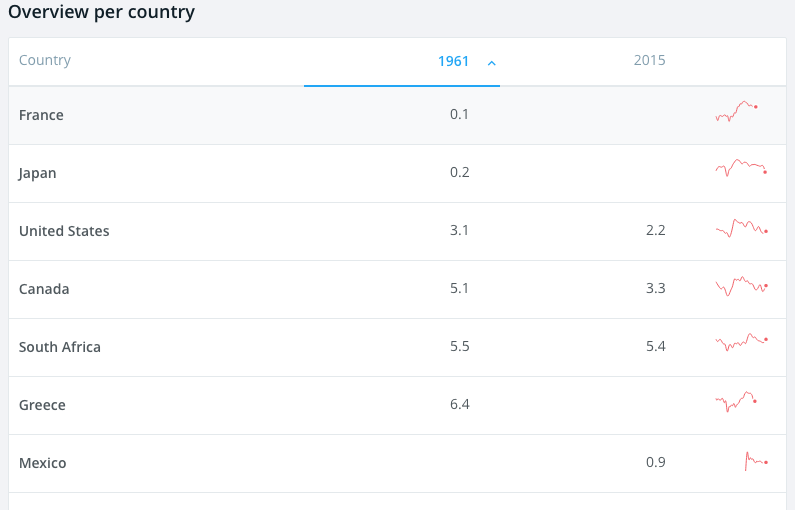# What is Real Interest Rate

The table below is the real interest rates in 1961 and 2015 for the various countries of the world. This data is made available thanks to IMF, International Financial Statistics and World Bank.Investors make investment decisions based on interest rates. Countries estimate interest rates in the coming years. Interest rates can be nominal and real. The difference between the two being inflation.

The nominal interest rate is the rate we see on loan products such as credit cards (credit card interest rates also include risk premium). The nominal rate is adjusted for inflation and the resulting interest rate is called real interest rate. Irving Fischer in his theory of interest defines real interest as:

Real interest rate = Nominal Interest Rate – Inflation

Real interest rates help measure the percentage return from an investment. Real interest rates inform investors about the economic climate of the country. High real interest rates are indicative of a stringent monetary policy and low real interest rates are indicative of a more accommodating monetary policy. If we look at the numbers on the chart the real interest rates in 2015 are either low or negative in order to enable a full economic recovery.

If real interest rates are high, investments move into saving and away from consumption. The converse is true when real interest rates are low.

Let us take a bond worth $100 with a one-year tenure and a interest rate of 5%. At the end of one- year you should get back$105. This is the return without accounting for inflation.

If we factor in inflation at say 2%, then the real rate of would be 3% and not 5%.